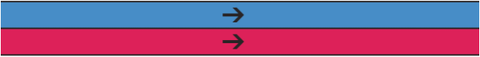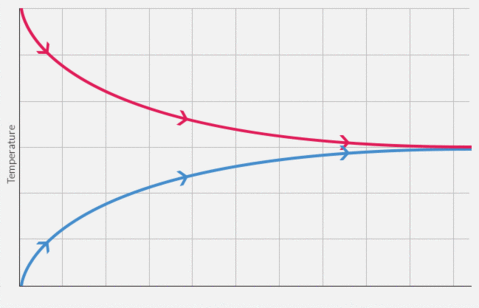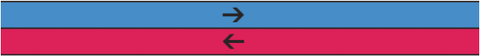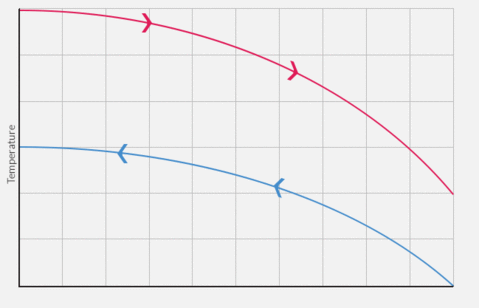You’ve had it happen, right? You hooked up your plate heat exchanger, it didn’t cool your wort nearly as much as you would have liked, and you thought it was broken. Then, after getting someone else to check it out, they point out that you hooked the hoses on the wrong way and are running it in parallel, not counterflow.

Oops.

Heat exchange is complex and confusing. I spent a long time reading up on the math and understanding how to explain counterflow vs parallel flow and I realized that the only real explanation would end up like this:And you would end up like this:So instead, I am going to try to explain the concept as simply as I can. No math.

Now, first thing to understand: the amount of heat transferred per second increases and decreases as the heat differential (the difference in temperature between the hot and the cold substance) increases or decreases. So two substances with a 100 degree differential will cool/warm faster (in ° per minute) than two with a 10 degree differential.

## Parallel Flow

So let's take an absurdly simplified example. Let’s assume we have a perfectly efficient, infinitely long heat exchanger with the same substance on each side, but one enters at 100 F and the other at 0 F. They both exit at 50 F (or very, very near that).The cooling relationship is logarithmic. What is happening is that as fluid enters the heat exchanger there is a 100° difference between the two, so they cool at a high rate. However, as the temperature differential drops, the speed at which heat exchanges also drops. This results in a temperature graph that looks more like this:You can see that using linear flow, the most you can ever heat or cool to is the midpoint of the differential. That means that given an infinitely long heat exchanger and 0° and 100° fluids, the eventual temperature of both the hot and the cold will reach 50°.

This is fairly efficient at first but quickly runs out of steam (pun intended). It limits the maximum temperature change to 50% of the differential.

## Counterflow

Counterflow just takes one stream and reverses it.This means that as the hot fluid enters from the right, it cools against the already warmed up cold fluid from the left. The temperature differential is nowhere near as high as at the start of the parallel heat exchanger. However, as the temperature of the warm fluids drops, it gets exposed to even colder cool fluid.This means that while the heat differential is never as large as it is at the beginning of parallel flow system, over the course of cooling, it stays large, resulting in total heat exchange much greater than a parallel model - with a theoretical maximum cooling/heating of 100% of the differential (in an infinitely long system).

This results in far more efficient cooling - which explains why counterflow is by far the dominant way to operate a heat exchanger.You’re right, the time label makes that graph all kinds of nonsense (why is the direction of time different for each line?).

A more accurate label would be “position in heat exchanger”.I believe that your x-axis label for your counterflow plot is incorrect. If the x-axis really was time then the temperature of the cold fluid would be increasing as time increases.